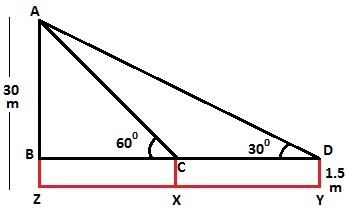# A 1.5 m tall boy is standing at some distance from a 30 m tall building. The angle of elevation from his eyes to the top of the building increases from 30° to 60° as he walks towards the building. Find the distance he walked towards the building.

Let the boy initially stand at point Y with inclination 30° and then he approaches the building to the point X with inclination 60°.To Find: The distance boy walked towards the building i.e. XY

From the diagram,, XY = CD.

Height of the building = AZ = 30 m.

AB = AZ – BZ = 30 – 1.5 = 28.5

Measure of AB is 28.5 m

In right ΔABD,

tan 30° = AB/BD 1/√3 = 28.5/BD

BD = 28.5√3 m

Also, In right ΔABC,

tan 60° = AB/BC √3 = 28.5/BC

BC = 28.5/√3 = 28.5√3/3

So, the length of BC is 28.5√3/3 m.

XY = CD = BD – BC = (28.5√3-28.5√3/3)

= 28.5√3(1-1/3)

= 28.5√3 × 2/3

= 57/√3 m.

Thus, the distance boy walked towards the building is 57/√3 m.(10)(19)